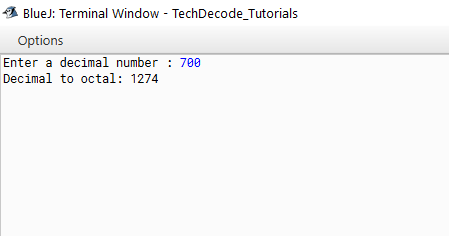# How to Convert Decimal to Octal in Java

The Conversion Of Decimal to Octal in Java is the conversion of number bases. Let us know about both number types before we start.

This is an eight-base system that employs numbers ranging from 0 to 7. Apart from Binary, Decimal, and Hexadecimal Numbers, it is one of the classes of number systems. The octal symbol is used to represent numbers with an eight-digit base. The relevance and uses of octal numbers are numerous. One of the most popular applications is in computer fundamentals. An Octal number system has an ‘eight’ as its foundation and employs numbers ranging from 0 to 7, i.e., 0, 1, 2, 3, 4, 5, 6, and 7. To further explain the notion, we may use an example. Any number with a base of eight, such as 24(8), 109(8), or 55(8), is known as an octal number.

Decimal is a term that describes the base-10 number system, probably the most commonly used number system. The decimal number system consists of ten single-digit numbers: 0, 1, 2, 3, 4, 5, 6, 7, 8, and 9. The number after 9 is 10.

## What’s the Approach?

• Create A `Scanner Class` object using `Scanner sc=new Scanner (System.in);`

• Accept the user’s input and store it in an `integer variable named dec.`

• Convert it to octal using `Integer.toOctalString(dec);`

• Print the `Octal` String.

Also Read: How To Convert Boolean To String in Java

### Java Program to Convert Decimal to Octal:

```/*

* TechDecode Tutorials

*

* How to Convert Decimal to Octal

*

*/

import java.util.*;

class Dec_To_Oct

{

public static void main(String args[])

{

//Creating scanner Class Object

Scanner sc = new Scanner( System.in );

//Accpeting user's input.

System.out.print("Enter a decimal number : ");

int dec =sc.nextInt();

//Converting

String oct= Integer.toOctalString(dec);

System.out.println("Decimal to octal: " + oct);

}

}```

Output: